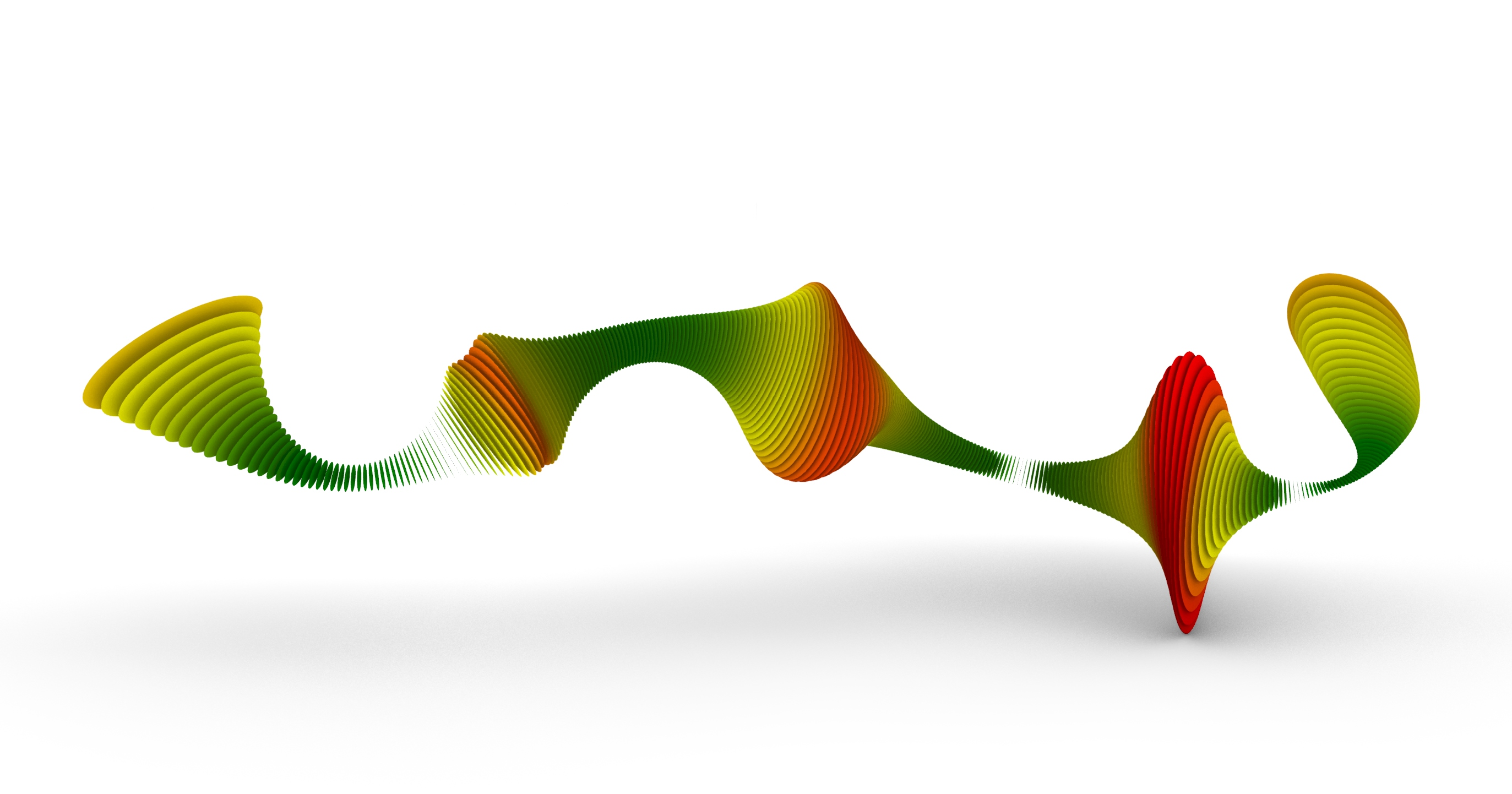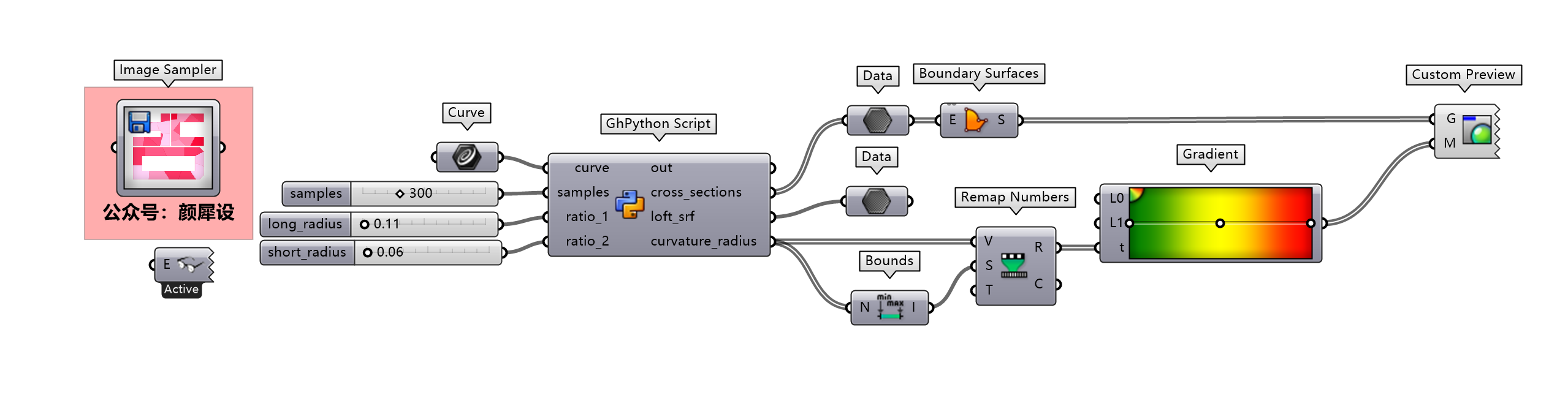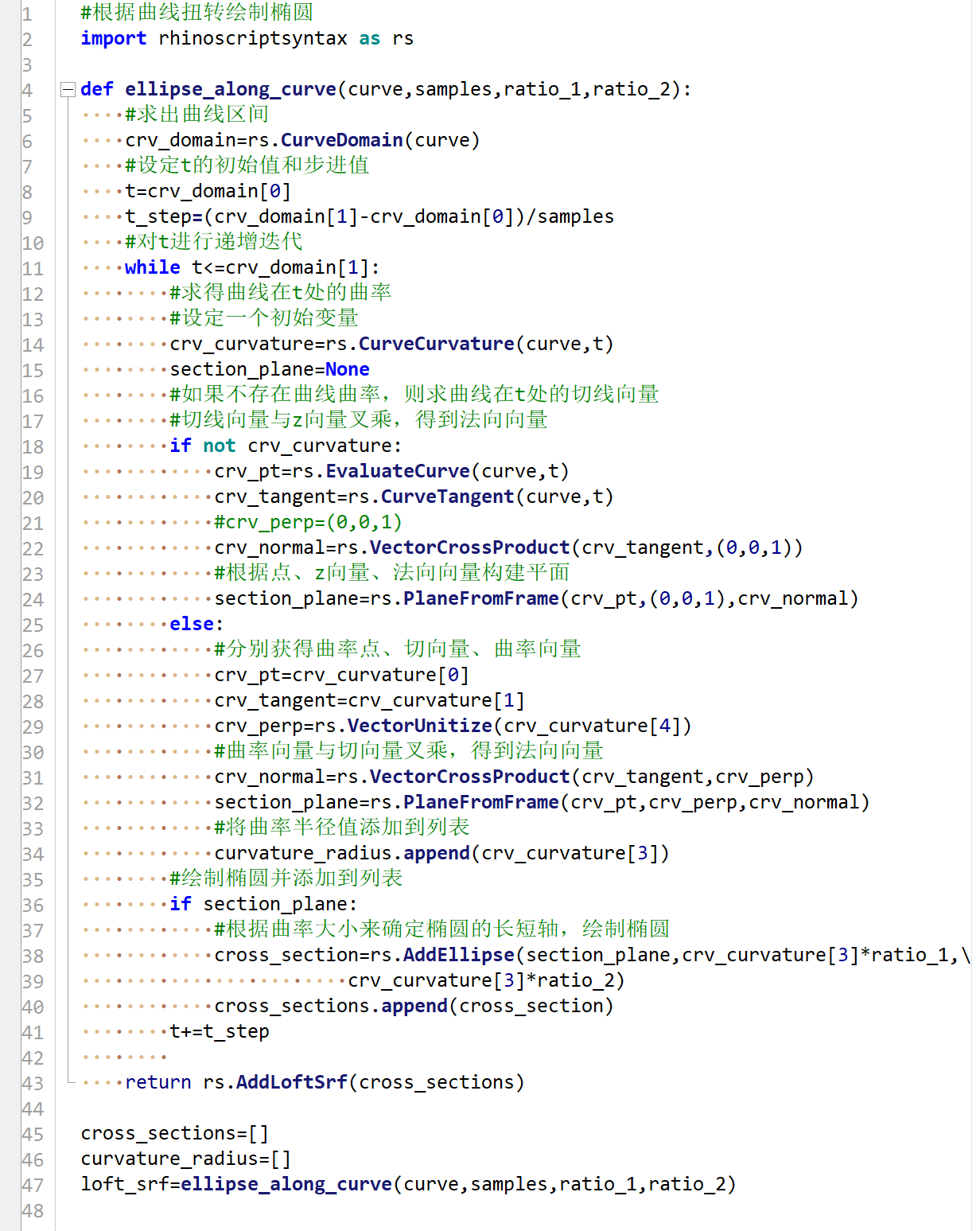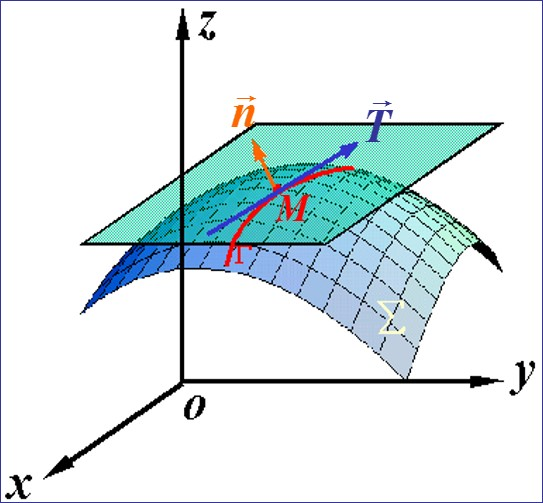• 今天继续聊rhinopython101里的案例，根据曲线的曲率向量和切向量绘制椭圆，其实就是通过椭圆的走向来反应曲线的扭转情况。 1.求出曲线的区间值； 2.设定t值初始值为曲线起点处，根据采样点数，求得曲线t值的步进值；...
今天继续聊rhinopython101里的案例，根据曲线的曲率向量和切向量绘制椭圆，其实就是通过椭圆的走向来反应曲线的扭转情况。
1.求出曲线的区间值；
2.设定t值初始值为曲线起点处，根据采样点数，求得曲线t值的步进值；
3.利用while循环对t值进行迭代，分别求得每个t值处的取虑点、切向量、曲率向量，曲率向量和切向量叉乘，可以得到法向量；
4.根据法向量和曲率向量构建平面，在平面上绘制椭圆；
5.为了最后对曲率椭圆进行着色，这里将曲率半径也作为参数列表输出，用来映射颜色值。
#ghpython展开全文• 开学已经是第二周了，我的《微分几何》也上课两周了，进度比较慢，现在才讲到平面曲线的曲率。在平面曲线$\boldsymbol{t}(t)=(x(t),y(t))$某点上可以找出单位切向量。$$\boldsymbol{t}=\left(\frac{dx}{ds},\frac{dy... 开学已经是第二周了，我的《微分几何》也上课两周了，进度比较慢，现在才讲到平面曲线的曲率。在平面曲线\boldsymbol{t}(t)=(x(t),y(t))某点上可以找出单位切向量。$$\boldsymbol{t}=\left(\frac{dx}{ds},\frac{dy}{ds}\right)$$其中ds^2 =dx^2+dy^2，将这个向量逆时针旋转90度之后，就可以定义相应的单位法向量\boldsymbol{n}，即\boldsymbol{t}\cdot\boldsymbol{n}=0。常规写法让我们用弧长s作为参数来描述曲线方程，\boldsymbol{t}(s)=(x(s),y(s))，函数上的一点表示对s求导。那么我们来考虑\dot{\boldsymbol{t}}，由于\boldsymbol{t}^2=1，对s求导得到$$\boldsymbol{t}\cdot\dot{\boldsymbol{t}}=0$$也就是说\dot{\boldsymbol{t}}与\boldsymbol{t}垂直，由于只是在平面上，所以\dot{\boldsymbol{t}}与\boldsymbol{n}平行。即$$\dot{\boldsymbol{t}}=\kappa \boldsymbol{n}$$类似地，有\dot{\boldsymbol{n}}与\boldsymbol{t}平行。并且对\boldsymbol{t}\cdot\boldsymbol{n}=0求导得到$$\dot{\boldsymbol{t}}\cdot\boldsymbol{n}+\boldsymbol{t}\cdot\dot{\boldsymbol{n}}=0$$将\dot{\boldsymbol{t}}=\kappa \boldsymbol{n}代入上式得到$$\dot{\boldsymbol{n}}=-\kappa \boldsymbol{t}$$\kappa被称为曲线在该点的曲率。复数表示以上是教科书的标准写法，但事实上，研究平面曲线的最方便的工具还是复数。将\boldsymbol{r}(s)用一个带参数的复数表示z(s)，那么上面的两式可以写成更简洁的一个式子$$\ddot{z}(s)=i\kappa (s) \dot{z}(s) $$这样写的好处还在于，任意给出曲率函数\kappa (s) ，我们就可以求出对应的曲线$$z(s)=\int e^{i\int \kappa (s)ds}ds $$这是简洁而有效的。另外，不妨设dz=ds e^{i\phi}，那么$$\dot{z}=e^{i\phi}$$自然地$$\ddot{z}=e^{i\phi}\left(i\dot{\phi}\right)$$所以曲率可以表示为$$\kappa=\dot{\phi}$$各种坐标利用它可以很方便地推导出各种坐标系下的曲率表达式，如曲线为一般的参数方程(x(t),y(t))时，用函数加一撇表示对t求导，有ds=\sqrt{x'(t)^2+y'(t)^2}dt,\phi=\arctan\left(\frac{y'(t)}{x'(t)}\right)，那么$$\frac{d\phi}{ds}=\frac{\frac{y''(t)}{x'(t)}-\frac{y'(t)x''(t)}{[x'(t)]^2}}{1+\left(\frac{y'(t)}{x'(t)}\right)^2}\div \left(\frac{ds}{dt}\right)$$代入整理易得$$\kappa=\frac{y''(t) x'(t)-x''(t) y'(t)}{[x'(t)^2+y'(t)^2]^{3/2}}$$在极坐标下，设r=f(\theta)，则z=f(\theta)e^{i\theta}，那么$$dz=\left(\frac{d f}{d \theta}+i f\right)e^{i\theta}d\theta$$所以$$ds=\sqrt{f^2+\left(\frac{d f}{d \theta}\right)^2}d\theta$$而\phi=\arctan\frac{f}{\left(\frac{d f}{d \theta}\right)}+\theta，那么$$\frac{d\phi}{ds}=\left[\frac{1-\left(\frac{d^2 f}{d \theta^2}\right) f/\left(\frac{d f}{d \theta}\right)^2}{1+f^2/\left(\frac{d f}{d \theta}\right)^2}+1\right]\div \left(\frac{d s}{d \theta}\right)$$代入整理得$$\kappa=\frac{2\left(\frac{d f}{d \theta}\right)^2+f^2-\left(\frac{d^2 f}{d \theta^2}\right)f}{\left[\left(\frac{d f}{d \theta}\right)^2+f^2\right]^{3/2}}三维空间有没有类似方便的东西呢？我也正在思考^_^更详细的转载事宜请参考：《科学空间FAQ》如果您还有什么疑惑或建议，欢迎在下方评论区继续讨论。如果您觉得本文还不错，欢迎分享/打赏本文。打赏并非要从中获得收益，而是希望知道科学空间获得了多少读者的真心关注。当然，如果你无视它，也不会影响你的阅读。再次表示欢迎和感谢！打赏微信打赏支付宝打赏因为网站后台对打赏并无记录，因此欢迎在打赏时候备注留言。你还可以点击这里或在下方评论区留言来告知你的建议或需求。如果您需要引用本文，请参考：苏剑林. (2014, Mar 04). 《平面曲线的曲率的复数表示 》[Blog post]. Retrieved from https://spaces.ac.cn/archives/2403
展开全文• 对于定义域是平面的函数 f(x,y)f(x,y)f(x,y), 我们定义 等高线(level curve, contour curve) f(x,y)=kf(x,y)=kf(x,y)=k 为二维曲线, 可以由 w=kw=kw=k 得到, 其中 kkk 是一个常数. 对于定义域是三维函数f(x,y,z)f...
多个变量的函数

比如, $w=f(x,y)$是三维空间中的曲面, $w=f(x,y,z)$是四维空间中的曲面.
对于定义域是平面的函数 $f(x,y)$, 我们定义 等高线(level curve, contour curve) $f(x,y)=k$ 为二维曲线, 可以由 $w=k$ 得到, 其中 $k$ 是一个常数.
对于定义域是三维的函数$f(x,y,z)$, 我们相应的可以定义 等高面(level surfaces) $f(x,y,z)=k$.
等高线是$z=f(x,y)$和$z=k$两个曲面的交叉, 另一个定义traces是$z=f(x,y)$与$x=k$ 或 $y=k$的交叉.

See https://tutorial.math.lamar.edu/classes/calciii/MultiVrbleFcns.aspx#LevelCurve example 4 for more visual illustrations.
偏导数
偏导数的定义很简单,比如说对于一个函数 $f(x_1,x_2,...,x_n)$, $f$ 对 $x_i$ 的偏导即为
$\frac{\partial f}{\partial x_i}=\lim_{\alpha\rightarrow 0}\frac{f(x_1,x_2, ..., x_i+\alpha,...,x_n)-f(x_1,x_2,...,x_n)}{\alpha}$
即,当沿着 $[0,...,\alpha,...,0]$的方向移动时, $f$的变化快慢. 注意对于偏导数而言, 方向固定为$[0,...,\alpha,...,0]$这是在$\mathbb{R}^n$中移动的. 如果$n=2$,那实际上方向是二维的,就只在水平面上移动, 这个偏导数的大小就是沿着给定方向变化的快慢.
方向导数
那么如果我们想看$f$沿着$\mathbb{R}^n$任一方向的变化快慢 (而不仅仅是bases的方向), 就得用到方向导数了. 定义如下:
$f(x_1,x_2,...,x_n)$ 沿着方向 $\bm{t}=(t_1,t_2,...,t_n)^\top$ (通常$\bm{t}$模长为1) 的方向导数为
$D_\bm{t} f(x_1,x_2,...,x_n)=\lim_{\alpha\rightarrow 0}\frac{f(x_1+t_1\alpha,x_2+t_2\alpha, ...,x_n+t_n\alpha)-f(x_1,x_2,...,x_n)}{\alpha}$
$=\frac{\partial f}{\partial x_1}t_1+\frac{\partial f}{\partial x_2}t_2+...+\frac{\partial f}{\partial x_n}t_n$
$\nabla f=\left[\frac{\partial f}{\partial x_1}, \frac{\partial f}{\partial x_2}, ...,\frac{\partial f}{\partial x_n}\right]^\top$
$f(\bm{x})$沿着 $\bm{t}$ 的方向导数可以更简洁的写为
$D_t f(\bm{x})=(\nabla f)^\top \cdot \bm{t}$
where $\bm{x}, \bm{t}\ \in\mathbb{R}^n$.
注意:梯度gradient是一个方向(且只在$\mathbb{R}^n$上), 方向导数则是一个数(沿着某一方向的变化率).

当 $\bm{t}$和$\nabla f$同向时,方向导数最大, 函数上升速度最快; 反向时,方向导数最小(负的),
函数下降速度最快.
对于$f(x_1,x_2,..,x_n)$, 它在$\bm{t}=(t_1,t_2,...,t_n)$处的梯度与等高面$f(x_1,x_2,..,x_n)=k$正交,
其中$f(x_1,x_2,..,x_n)=k$经过$\bm{t}$.
=> 令等高面为 $\mathcal{S}$,  $\bm{t}$在$\mathcal{S}$上, 且$\mathcal{S}$上的任意一条经过$\bm{t}$的curve都与 $\nabla f(x_1,x_2,..,x_n)$ 正交

曲面的两种写法
好,最后我们来看看高维曲面的切平面和法向量.
首先, 我们知道一个曲面有两种写法
[第一种写法]
$z=f(x_1,x_2,...,x_n)$
第一种写法表示 $(x_1,x_2,...,x_n)$ 的定义域在一个 $n$ 维空间中, 自变量只能在这个空间中到处跑然后$z$取得不同的值. 所以当我们算任意一点的 gradient 时 – 这里的任意一点指的是$(x_1,x_2,...,x_n)$, 一般是optimization 问题的定义域
$\nabla f(x_1,x_2,...,x_n)=\left[ \frac{\partial f}{\partial x_1},\frac{\partial f}{\partial x_2} , \cdots, \frac{\partial f}{\partial x_n} \right]^\top$
他表示的是一个 $n$维空间的向量, 在任意一点都指示着接下来往哪走是函数上升最快的方向.
[第二种写法]
$F(x_1,x_2,...,x_n,z)=f(x_1,x_2,...,x_n)-z=0$
不同于第一种写法,这种写法是将 $f(x_1,x_2,...,x_n)$ 描述为 一个 $n+1$ 维空间中一个图形 $F(x_1,x_2,...,x_n,z)$ 的一个截面: 若令 $w=F(x_1,x_2,...,x_n,z)$, 那么 $f(x_1,x_2,...,x_n)$ 是 $w= F(x_1,x_2,...,x_n,z)$ 和 $w=0$ 的截面.
若对 $F$ 求gradient, 那么这个 gradient 实际上是一个 $n+1$ 维的向量 – “任意一点的梯度” 这句话中的任意一点是 $(x_1,x_2,...,x_n,z)$
$\nabla F(x_1,x_2,...,x_n,z)=\left[ \frac{\partial F}{\partial x_1},\frac{\partial F}{\partial x_2} , \cdots, \frac{\partial F}{\partial x_n}, \frac{\partial F}{\partial z} \right]^\top$
$= \left[ \frac{\partial f}{\partial x_1},\frac{\partial f}{\partial x_2} , \cdots, \frac{\partial f}{\partial x_n}, -1 \right]^\top$
注意,这个梯度的前 $n$ 项与 第一种写法下的梯度 $\nabla f(x_1,x_2,...,x_n)$ 是完全一致的, 是同一个方向, 因此,

$\nabla f(x_1,x_2,...,x_n)$是 $\nabla F(x_1,x_2,...,x_n,z)$ 在 $\mathbb{R}^n$ 上的投影

切平面, 法向量, 法线
现在让我们想想, 对于$f$上任意一点 $(t_1,t_2,...,t_n, t_z)$, where $t_z=f(t_1,t_2,...,t_n)$, 我们怎么写出它的切平面 tangent (hyper) plane) 和 法线 normal line. 显然, 如果我们用第一种写法, 是得不到的因为他只限于$n$ 维空间. 而切平面 和 法线 实际上都是 $n+1$ 维空间的. 所以我们采用第二种写法.
已知:

=> 这个可以由上一节最后的结论推出: $n+1$ 维函数 $F$ 令 $k=0$.
曲面上任意一点的切平面由 所有与法向量垂直的高维直线 构成.

则有: 切平面
$\left[\nabla F(\bm{x},z)\right]^\top [x_1-t_1,x_2-t_2,...,x_n-t_n, z-t_z]^\top= 0$
$\left[ \frac{\partial f}{\partial x_1},\frac{\partial f}{\partial x_2} , \cdots, \frac{\partial f}{\partial x_n}, -1 \right] [x_1-t_1,x_2-t_2,...,x_n-t_n, z-t_z]^\top=0$
从这个式子中,我们也能得到第一种表达方式下的切平面方程. Let $\bm{x} = (x_1,x_2,...,x_n, z)^\top$, $\bm{t} = (t_1,t_2,...,t_n, t_z)^\top$,
$[\nabla f(x_1,x_2,...,x_n) ]^\top (\bm{x}-\bm{t}) + f(t_1,t_2,...,t_n) = z$
最后我们来求法线. 已知,

法向量是 $\nabla F(x_1,x_2,...,x_n,z)$
法线过点$(t_1,t_2,...,t_n, t_z)$

hyperplane中的一条线可以用参数方程表示: 任意一个向量, 比如$\nabla F(x_1,x_2,...,x_n,z)$, scale之后可以用来表示穿过原点的一条直线上的所有点, 若我们在这个向量上再加一个vector,就可以让他平行的移动了. 所以, 法线的参数方程为
$(t_1,t_2,...,t_n, t_z)+t\nabla F(x_1,x_2,...,x_n,z)$
Reference:


展开全文• 设t=t0时对应点M，那么M点处的切向量为： 切线方程为： M点处的法向量为：， 法线方程为： ，, å上过点M的任何曲线在该点的切线都在同一平面上，此平面称为在该点的切平面，切平面的方程为： ， 2.曲...
0 二维曲线

对于二维曲线上的某一个点，他的梯度组成的向量就是他的法向量，证：
因为二维曲线某一个点的曲线部分可以看成直线，设直线方程： ax+by+c=0$ax+by+c=0$$ax + by + c = 0$ 那么他的斜率为−ab$-\frac{a}{b}$$-\frac{a}{b}$, 该方程x方向与y方向的梯度分别为： (a,b)$\left(a,b\right)$$(a, b)$,这个向量的斜率为ba$\frac{b}{a}$$\frac{b}{a}$,两者相乘恰好是-1，因此证明某个点的梯度其实就是这个点所在那部分曲线的法线。

以下转自： 文章地址

（博文大部分取自于北科的课件，略加整理而成…）
1.曲面方程为隐式方程的情况：
光滑曲面方程形式为：在曲面上任意取一点M(x0,y0,z0),曲线方程为：设t=t0时对应点M，那么M点处的切向量为：切线方程为：M点处的法向量为：，
法线方程为：，,
å上过点M的任何曲线在该点的切线都在同一平面上，此平面称为在该点的切平面，切平面的方程为：，2.曲面方程为显式方程的情况：实例：展开全文• （博文大部分来自于北科的课件） 1.曲面方程为隐式方程的...不能全部为零，那么M点处的切向量为 ， 切线方程为： M点处的法向量为： ， 法线方程为： å 上过点 M 的任何曲线在该点的切线都在同一平面上，此
• PART 01 复平面上的曲线 参数方程与曲线的方向 定义 若平面点集可以表示为由区间 到复平面的连续映射下的像集，则该点集就称为一条平面曲线，该映射称为该曲线的一个参数方程．...曲线的切向量复变函数
• 曲面法向量 在求曲面切平面时候，需要知道曲面法向量，曲面法向量是怎么求得呢？ 先给出结论：曲面法向量是三元函数梯度，曲线向量是二元函数梯度
•  曲线的切向量：切线的方向向量称为曲线的切向量．向量 T=(j¢(t0),y¢(t0),w¢(t0)) 就是曲线G在点M0处的一个切向量． <br />  法平面：通过点M0而与切线垂直的平面称为曲线G在点M0处...
• 4. 向量值函数可导性（向量值函数导数、切向量、切线、法平面、光滑曲线） 5. 向量值函数物理意义（运动速度、速率、加速度） 6. 向量值函数求导法则 7....
• 空间曲线的切线与法平面求法设空间曲线的方程及其上的一点对应于则曲线在处的切线方程为:切向量为:法平面方程为:2. 例题1.求圆柱螺旋线在对应点处的切线方程和法平面方程.课堂索引：8.6多元函数微分学的几何应用(1)...
• 然后得到各边界点切向量垂直平面与边界交叉点, 并与对应边界点构成交叉向量; 利用内法向量和交叉向量加权和计算边界点生长方向, 根据原始点云密度确定生长步长, 从而进行修补; 最后, 利用拉普拉斯平滑算法...
• 20多年大学授课一线经验；数学博士、硕导、教授；考研阅卷组组长；授课风格幽默诙谐，通俗易懂，简单好记...本章目录3.1、向量内积、外积3.2、空间平面与直线3.3、空间曲线的切线与法平面3.4、空间曲线的切平面与法...
• 1、切线 ——过曲线上两点N,M的直线NM...7、曲率——针对曲线上某个点的切线方向角对弧长的转动率，曲率越大，表示曲线的弯曲程度越大。曲率的倒数就是曲率半径； 8、挠率——它的绝对值度量了曲线上邻近两点的次法向量
• 偏导数在几何上的应用问题引入曲面的切平面和法线切平面方程与法线方程例1 球面的切平面与法线方程例2 旋转抛物面的切平面与法线方程参数曲面的切平面参数方程的法向量例4方程组所确定的空间曲线的切线切向量例5 ...算法
• 空间曲线，空间曲面 空间曲线的两种方式：①参数式②两面相交式 空间曲线一点的切线：①参数式：对x，y，z求t的偏导，②两面相交式：两个方程对x求导，解出两个偏导，切线： ...空间曲面的切平面方程： ...
• 曲率是曲线的单位矢对弧长的转动率，表示曲线...由于平面曲线的次法向量处处与平面垂直，所以平面曲线挠率处处为零。也就是发生弯曲，不扭曲。 而对于三维曲线，某一点曲率，挠率都不为零，同时发生弯曲和扭曲 ...
• 多元函数的几何应用 ...例如已知关于t的运动轨迹，其一阶求导的切向量切向量为速度向量，二阶求导为加速度向量。 空间曲线的切线和法平面 先求导向量，将导向量作为方向向量，利用点向式方程求得切线，与切线...多元函数 梯度 方向导数
• 曲线的学习思路一般是这样的：那么对于曲面其实我们也可以采取类似的思路，当然由于曲面与曲线的不同之处所以在整个线路上也有不同的地方：切平面鉴于我们已知了曲线的切向量，那么我们就从曲面上的曲线开始吧，曲面...
• 如果知道一条空间曲线的参数方程，那它相应的切向量就是参数方程的分量，分别求导数来作为切向量的分量 切平面的方程 例1 （五颗星） 例2（反面的例子看看就行了） 二元函数全微分的概念 可微判定 例3 例...算法
• ## 弗莱纳公式

千次阅读 2018-11-13 15:25:04
转自：... 弗莱纳公式    空间曲线的切向量 T，法向量 N 和副法向量 B；以及切向量和法向量张成的密切平面。 在向量微积分中，弗莱纳公式(Frenet–Serret 公式)用来描述...
• 一、一元向量值函数及其导数 二、空间曲线的切线和法平面 三、曲面的切平面和法线
• 正则曲线(切向量不为零)>>切线>>弧长（度量）>>活动标架>>曲率挠率>>基本公式>>基本定理 典例 正则曲面>>切平面>>度量(第一基本形式)>>活动标架>>局部...
• T称为切向量（Tangent），表示沿曲线运动方向单位向量；N称为法向量（Normal），表示当前曲线运动平面内垂直于单位向量；B称为当前运动平面的副法向量（Binormal），表示同时垂直于和单位向量。令r(t)为这个...算法
• 第四讲 向量代数、多元函数微分与空间解析几何一、理论要求 1.向量代数 理解向量的概念(单位向量、方向余弦、模) 了解两个向量平行、垂直...会用Lagrange乘数法求极值 掌握曲线的切线与法平面、曲面的切平面与法线的...
• 高数：首先，这一节中的几个重要知识点有：一、求空间曲线的切向量（进而求切线方程与法平面）：主要有以下三种形式：①、给出参数方程形式（x=x（t），y=。。。，z=。。。）： 三个参数方程分别对自变量t求导即得...
• 多元函数微分学的几何应用主要是讲述空间向量与微分学的融合，包括：空间曲线的切线和空间曲面的切平面。如果将本文和之前的“空间向量”一文结合起来看，你会发现多元函数微分学与空间向量结合后的神奇。 一、...
• 1.4Botlquet公式、平面曲线相对曲率 1.5曲线论的基本定理 1.6曲率圆、渐缩线、渐伸线 1.7曲线的整体性质（4顶点定理、Minkowski定理、Fenchel定理） 第2章Rn中k维Cr曲面的局部性质 2.1曲面的参数表示、切向量、法...
• 曲线的切向量定义为曲线的求导，也就是曲线的斜率。把这个向量旋转90°就是曲线在这个点的法向量。在参数方程中，比如x1=(u, u)T和x2=(u²，u²)T这两个参数方程描述的曲线在[0,1]上是完全一样的，也就是说一条...
• Method of Characteristics: How...由点乘为0得出向量与法向量垂直，向量位于曲面S的切平面内 寻找一个曲面S，S上的每个点处的切平面都有一个向量位于切平面上 转而寻找一条曲线C，C位于S上，C上的每个点处的斜率为
• 往曲面的切平面做投影，得到向量就是测地曲率向量，这个向量的大小就是测地曲率。所以从定义上看，测地曲率刻画了曲线在曲面内蕴的弯曲程度，而法曲率刻画了曲线在嵌入空间的弯曲程度。比如一张平面上的直线的测地...几何